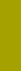﻿ Grain shapeSand Texture Sedimentology | Variables

Grain shape

Variable 4: Logarithmic Grain Shape Factor, LSF

Shape Factor of A. T. COREY (1949) and J. S. McNOWN + J. MALAIKA (1950) is used
(see Dispersity Variable 3, page 5 of this Manual)

LSF = -log2SF

LSF is a 3-character abbreviation of -log2SF;

this negative binary logarithm is consistent with PHI;

L stands for logarithm, SF stands for (Corey's) Shape Factor;

Our SedVar™ software can determine a grain shape distribution of equally sized homogeneous and inhomogeneous material, such as a narrow sieved single mineral fraction without a complicated optical measurement of grain shape. The settling rate will be interpreted in terms of shape factor for grains with a constant size and density. If the narrow sieved fraction consists not of one but several heavy minerals, then heavy liquid separation is unavoidable in order to obtain homogeneous subsamples, each with a narrow density interval of equally sized material. SedVar™ can provide a three dimensional plot of the results.

Accuracy of measurement of a shape distribution depends on factors 1 through 4 listed for density distribution (Dispersity variable 4, LRS, page 9), and on two additional ones:

5 Relative equivalence of density intervals: if expressed in g/cm3, the intervals must form a geometric series; expressed in binary logarithm, they must form an arithmetic series.

6 Narrowness of density intervals: expressed in g/cm3, they should be in ratio less than 1.1 or 0.9 for a low accuracy, or less than 1.05 or 0.95 for a higher accuracy; expressed in LSF notation, the interval should be smaller than 0.14 for a low accuracy, or smaller than 0.07 for a higher accuracy.

Note that the sedimentational method of shape determination loses its sensitivity (and validity) with decreasing grain size as shown in Fig. 5 of J. BREZINA (1979): PSI settling rate curves for various SF values converge toward finer particle size.

Therefore, the Shape Factor calculation is limited to coarser particles which provide sufficient SF resolution due to fairly wide PSI-difference. For example, the PSI-difference between SF 1.2 and 0.1 of equally sized and dense particles decreases 3-times (from 2.35 PSI to 0.77 PSI) if the particle size decreases 32-times (which corresponds to a 5 PHI difference); this is valid for every density. A density change causes an inversely proportional shift of a grain size square root:

 PSI-difference for equally sized grains with SF=1.2 and 0.1 2.35 PSI - Difference 0.77 PSI Difference density [g/cm3] mm = PHI mm = PHI 2.5 4.00 -2.0 0.125 +3.0 5.0 2.83 -1.5 0.088 +3.5 10.0 2.00 -1.0 0.063 +4.0 20.0 1.41 -0.5 0.044 +4.5

The PSI-LSF conversion implies a change of distribution type, similarly as the PSI-PHI conversion. This effect will be treated in a later edition of these pages.